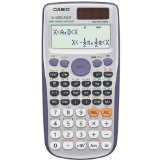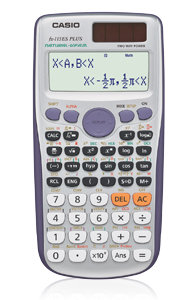### Casio fx-115ES PLUS Engineering/Scientific Calculator• Natural textbook display shows expressions and results exactly as they appear in the textbook
• Over 280 functions, including fractions, statistics, complex number calculations, base arithmetic, linear regression, standard deviation, computer science, and polar-rectangular conversions
• Multi-replay function allows you to backtrack step-by-step through calculations, where you can edit expressions and recalculate answers

#### CASIO fx-115ES Plus Scientific CalculatorCasio’s most advanced scientific calculator to date, has been designed and engineered for easy operation at any level. The Natural Display and enhanced features like displaying root forms of equations, finding derivatives, product notation templates, and advanced inequality functionality, makes the fx-115ES Plus extremely versatile and the perfect choice for high school and college students alike.

#### Suggested Courses

The fx-115ES Plus is designed to support all users in the following courses:

• All General Mathematics courses
• Pre-Algebra
• Algebra I & II
• Statistics
• Pre-Calculus
• Trignonmetry
• Chemistry
• Physics
• Engineering

#### Exam Allowance

The CASIO fx-115ES Plus scientific calculator is permitted for use on the PSAT/NMSQT, SAT and ACT college entrance exams, AP tests, and NCEES exams.

#### Hardware Features

The fx-115ES Plus is intuitive and easy to use as you progress through your coursework. It has two-way power, “Solar Plus with Battery Back-up”, so you’re always ready!

#### Large Display

Offers a large, multi-line display that allows for important multi-replay functionality.

#### Functionality & Key Features

Some of the functionality you will find in the fx-115ES Plus includes:

• Natural textbook display shows expressions and results exactly as they appear in the textbook
• Over 280 functions, including fractions, statistics, complex number calculations, base arithmetic, linear regression, standard deviation, computer science, and polar-rectangular conversions
• List-Based STAT Data Editor
• Table Function
• Matrix and Vector Calculations
• 40 Metric Conversions
• Multi-replay function allows you to backtrack step-by-step through calculations, where you can edit expressions and recalculate answers

List Price: \$ 17.99

Price: [wpramaprice asin=”B007W7SGLO”]

[wpramareviews asin=”B007W7SGLO”]

Related Products